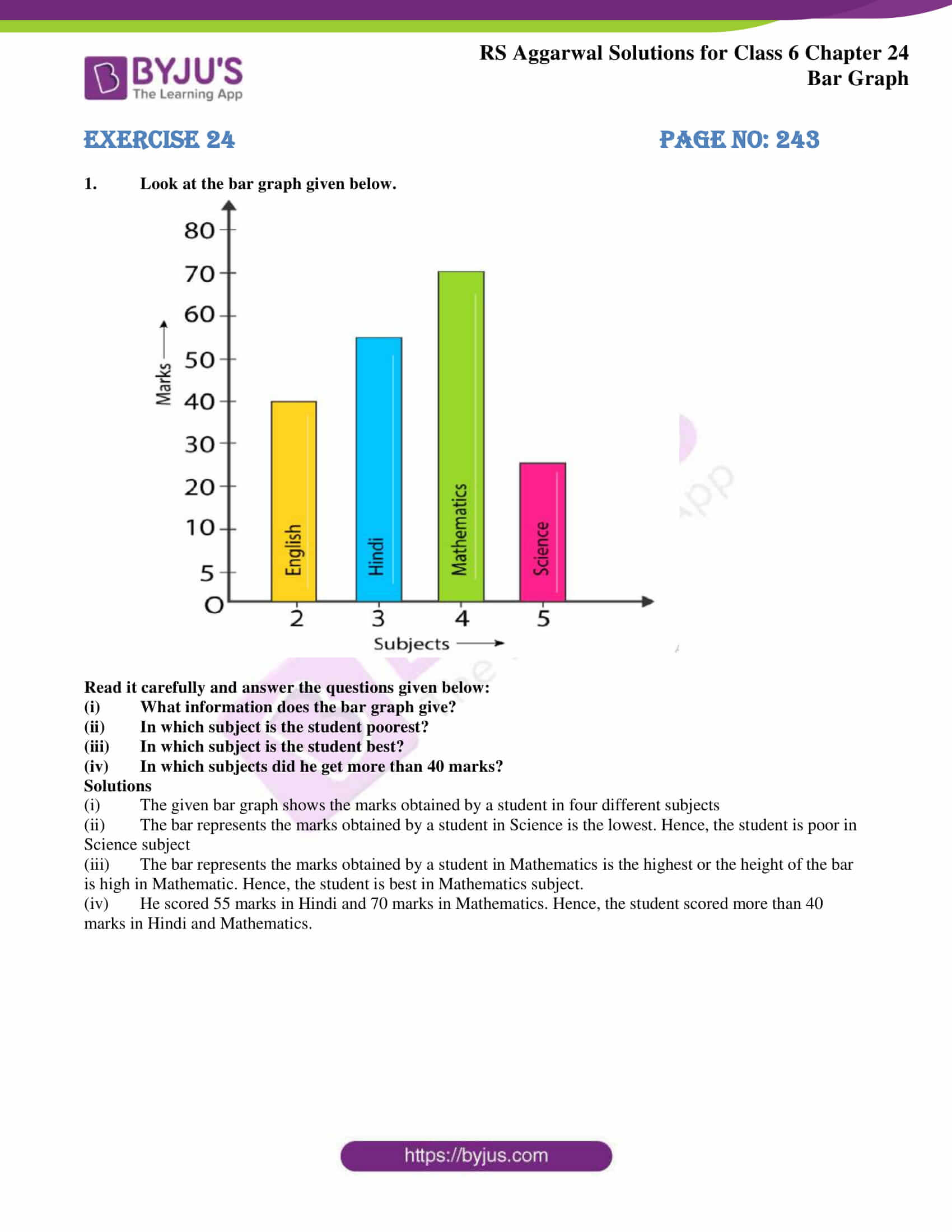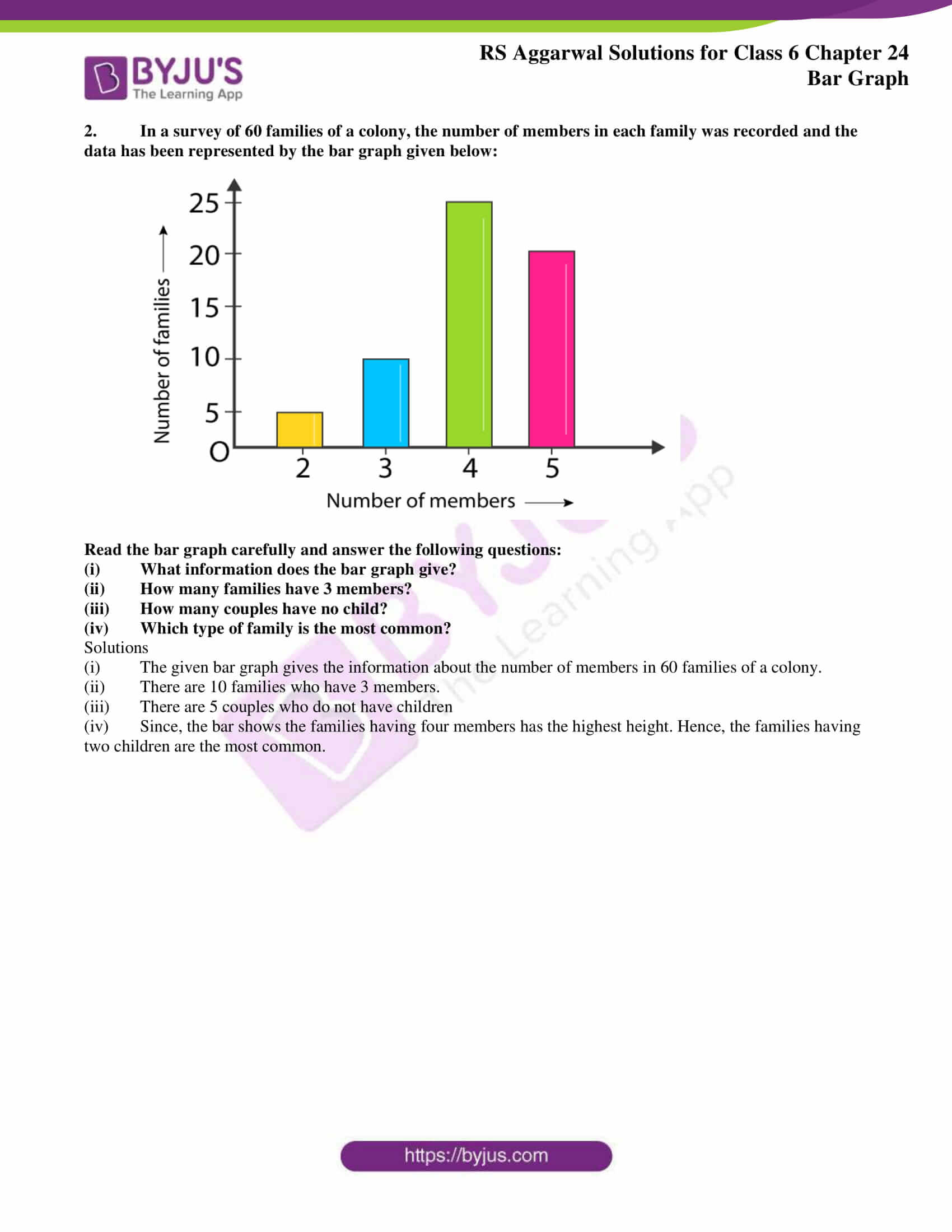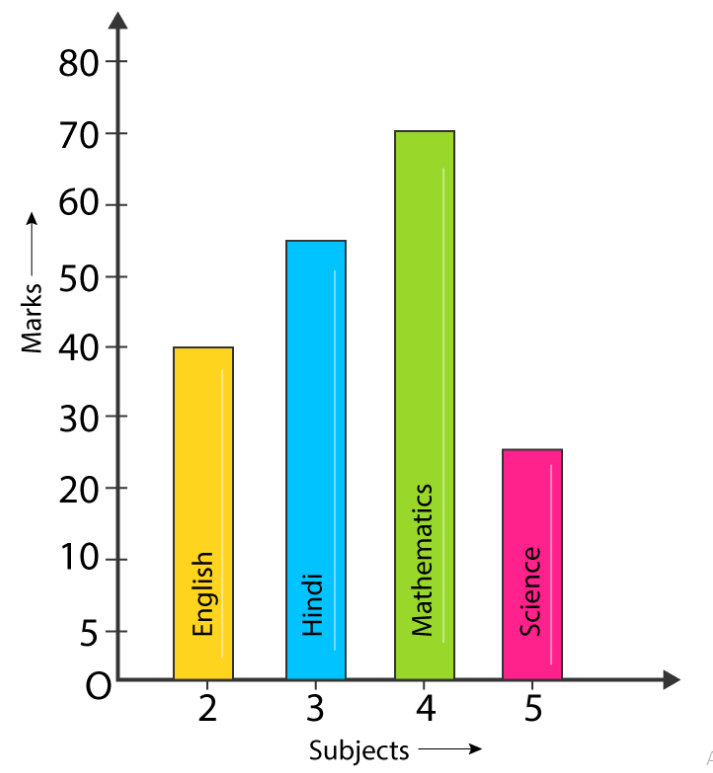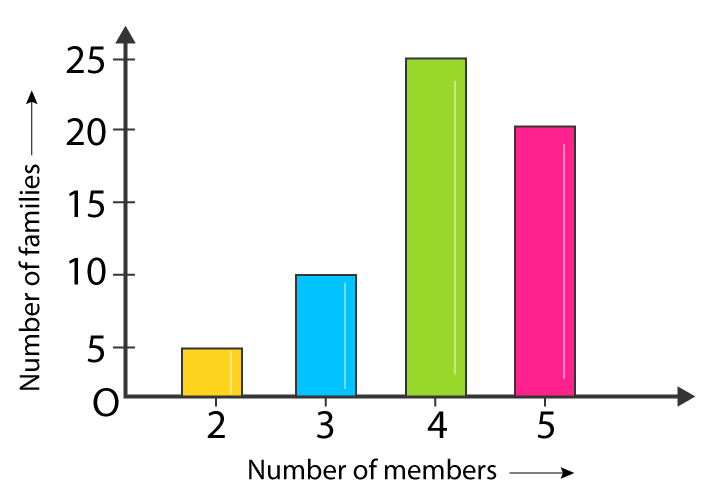# RS Aggarwal Solutions for Class 6 Maths Chapter 24 Bar Graph

RS Aggarwal Solutions for Class 6 Chapter 24 Bar Graph are given here in PDF format. Students who go through RS Aggarwal textbook questions will improve their knowledge and confidence in Mathematics. Students who want to learn how to read and draw Bar Graph are advised to practice RS Aggarwal textbook for Class 6 chapter 24. Solutions are provided in detail to help students in completing their homework without any obstacles.

The BYJU’S RS Aggarwal Solutions have been prepared by passionate Mathematics teachers in simple PDF format. These solutions are free to download. Download PDF of Class 6 chapter 24 in their respective links.

## Download PDF of RS Aggarwal Solutions for Class 6 Chapter 24 Bar Graph## Exercise 24 PAGE NO: 243

1. Look at the bar graph given below.(i) What information does the bar graph give?

(ii) In which subject is the student poorest?

(iii) In which subject is the student best?

(iv) In which subjects did he get more than 40 marks?

Solutions

(i) The given bar graph shows the marks obtained by a student in four different subjects

(ii) The bar represents the marks obtained by a student in Science is the lowest. Hence, the student is poor in Science subject

(iii) The bar represents the marks obtained by a student in Mathematics is the highest or the height of the bar is high in Mathematic. Hence, the student is best in Mathematics subject.

(iv) He scored 55 marks in Hindi and 70 marks in Mathematics. Hence, the student scored more than 40 marks in Hindi and Mathematics.

2. In a survey of 60 families of a colony, the number of members in each family was recorded and the data has been represented by the bar graph given below:(i) What information does the bar graph give?

(ii) How many families have 3 members?

(iii) How many couples have no child?

(iv) Which type of family is the most common?

Solutions

(i) The given bar graph gives the information about the number of members in 60 families of a colony.

(ii) There are 10 families who have 3 members.

(iii) There are 5 couples who do not have children

(iv) Since, the bar shows the families having four members has the highest height. Hence, the families having two children are the most common.

### RS Aggarwal Solutions for Class 6 Chapter 24 Bar Graph

Chapter 24 has 1 exercise. All questions of RS Aggarwal textbook have been solved step by step for students of class 6 who aspire to improve their skills in Mathematics. Let’s have a glance at the topic included in this chapter.

• Bar Graph
• Reading and interpretation of a Bar Graph

### Chapter Brief of RS Aggarwal Solutions for Class 6 Maths Chapter 24 Bar Graph

A graph which shows the values of different categories of data as rectangular bars with different lengths is known as a Bar Graph. Here students are taught how to read and draw a Bar Graph.